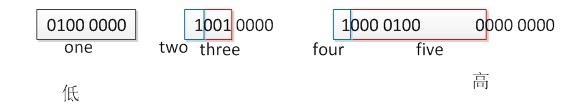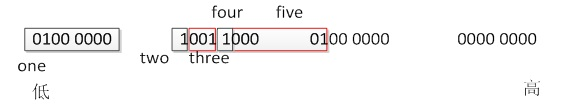/x86/Debian GNU/Linux/gcc

# 1 聯合體

## (2)用聯合體測平臺數據儲存是大端還是小端

``````//When this paltom uses litte address to be many bytes's address
//Test platom is litte or bit endian
void test_endian(void)
{
printf("\n--------------test_endian function------------------\n");
union one_test{
unsigned int i;
unsigned char ch;
}my_test={1};

printf("ch = %d\nhex dump of ch = 0x%x\n", my_test.ch, my_test.ch);

if(my_test.ch == 1){
printf("This platom uses litte endian\n");
}else{
printf("This platom uses big endian\n");
}
}
``````

my_test經初始化後，my_test.chmy_test.i的第一個位元組（表示整個變數的地址）內容相同。如果系統採用低地址來作為一個型別的地址int4個位元組，用4個位元組中的最低地址表示int變數的地址），那麼以上程式就能夠測試出系統採用的大端還是小端方式儲存資料。如果my_test.ch值為1則表示系統採用小端方式儲存資料，否則為大端方式。如果系統採用高地址來作為一個型別的地址，那麼my_test.ch值為1則表示系統採用大端方式儲存資料，否則為小端方式。

 --------------test_endian function------------------ address of i = 0xbff03cdc address of ch = 0xbff03cdc ch = 1 hex dump of ch = 0x1 This platom uses litte endian

# 2 位段

## (2)位段的儲存

``````//Test Bit-field's memory layout
void test_bit_field_layout(void)
{
printf("\n--------------test_bit_field_layout---------------\n");
union two_test{
struct bit_field{
unsigned int one	:8;
unsigned int two	:1;
unsigned int three	:3;
unsigned int 		:4;
unsigned int four	:1;
unsigned int five	:8;
}my_bit_field;
unsigned char byte;
}my_test={{2, 1, 4, 1, 16}};

printf("value of byte:\nbyte = %d\tbyte = %d\tbyte = %d\tbyte = %d\n",		\
my_test.byte, my_test.byte, my_test.byte, my_test.byte);
printf("\nhex dump of byte:\nbyte = 0x%x\tbyte = 0x%x\tbyte = 0x%x\tbyte = 0x%x\n",	\
my_test.byte, my_test.byte, my_test.byte, my_test.byte);
}
``````

 --------------test_bit_field_layout--------------- value of byte: byte = 2byte = 9byte = 33byte = 0 hex dump of byte: byte = 0x2    byte = 0x9    byte = 0x21 byte = 0x0--------------test_bit_field_layout--------------- value of byte: byte = 2byte = 25byte = 2byte = 0 hex dump of byte: byte = 0x2    byte = 0x19 byte = 0x2 byte = 0x0CNote Over.

.